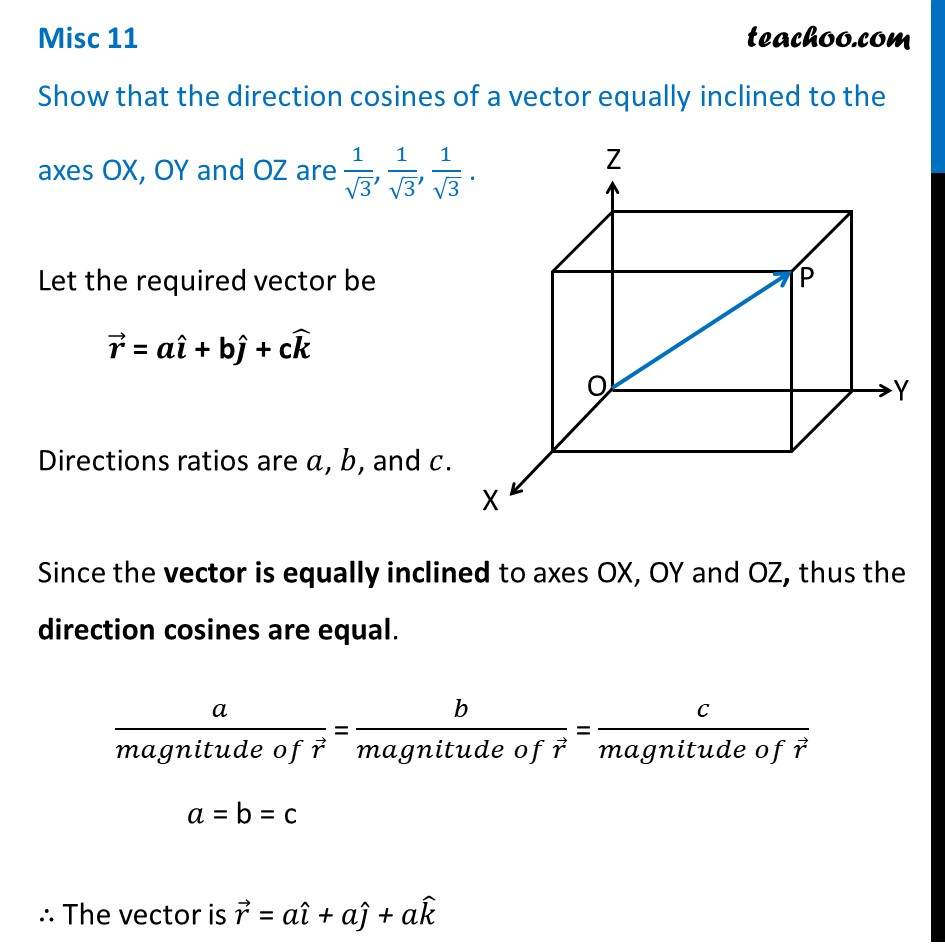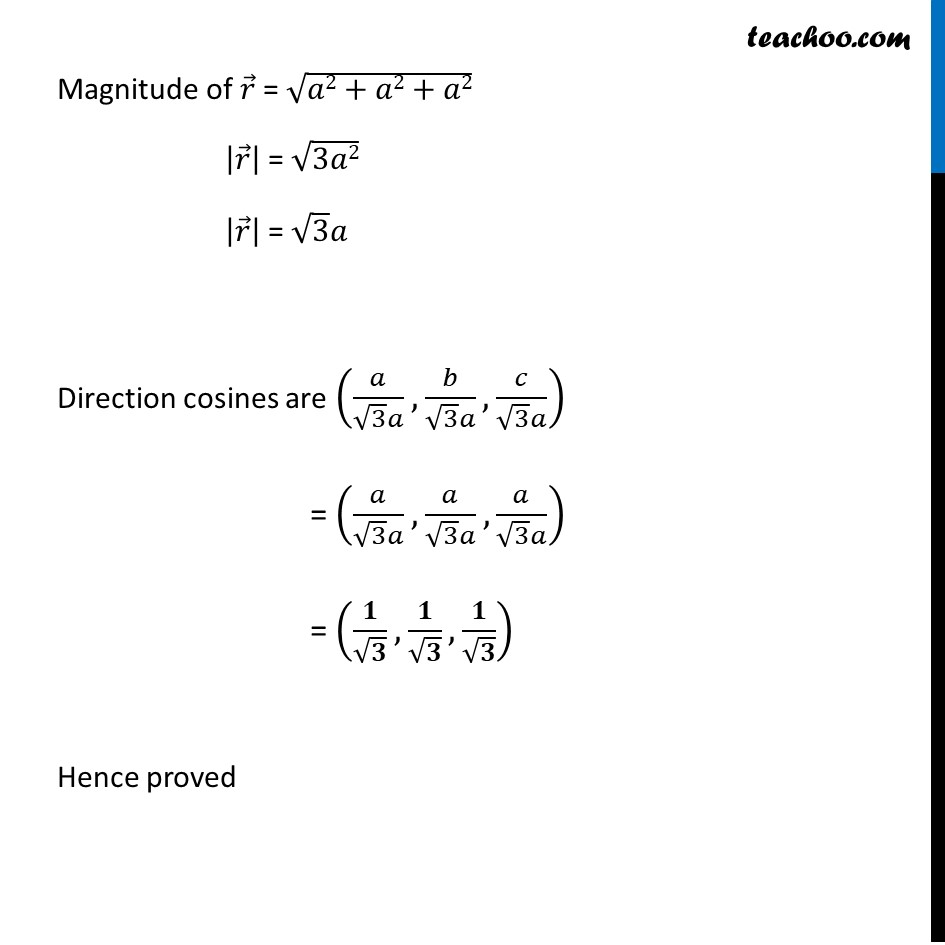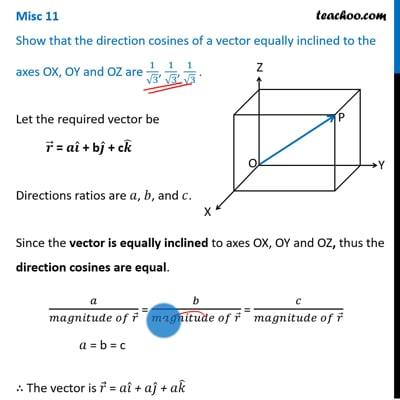Miscellaneous

Chapter 10 Class 12 Vector Algebra
Serial order wiseThis video is only available for Teachoo black users

Solve all your doubts with Teachoo Black (new monthly pack available now!)

### Transcript

Misc 11 Show that the direction cosines of a vector equally inclined to the axes OX, OY and OZ are 1/√3, 1/√3, 1/√3 . Let the required vector be 𝒓 ⃗ = 𝒂𝒊 ̂ + b𝒋 ̂ + c𝒌 ̂ Directions ratios are 𝑎, 𝑏, and 𝑐. Since the vector is equally inclined to axes OX, OY and OZ, thus the direction cosines are equal. 𝑎/(𝑚𝑎𝑔𝑛𝑖𝑡𝑢𝑑𝑒 𝑜𝑓 𝑟 ⃗ ) = 𝑏/(𝑚𝑎𝑔𝑛𝑖𝑡𝑢𝑑𝑒 𝑜𝑓 𝑟 ⃗ ) = 𝑐/(𝑚𝑎𝑔𝑛𝑖𝑡𝑢𝑑𝑒 𝑜𝑓 𝑟 ⃗ ) 𝑎 = b = c ∴ The vector is 𝑟 ⃗ = 𝑎𝑖 ̂ + 𝑎𝑗 ̂ + 𝑎𝑘 ̂ Magnitude of 𝑟 ⃗ = √(𝑎2+𝑎2+𝑎2) |𝑟 ⃗ | = √3𝑎2 |𝑟 ⃗ | = √3 𝑎 Direction cosines are (𝑎/(√3 𝑎),𝑏/(√3 𝑎),𝑐/(√3 𝑎)) = (𝑎/(√3 𝑎),𝑎/(√3 𝑎),𝑎/(√3 𝑎)) = (𝟏/√𝟑,𝟏/√𝟑,𝟏/√𝟑) Hence proved